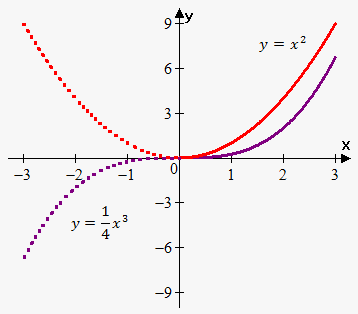# Even Odd Function

If f(x)=f(-x) for every x in the domain of f then f is an even function.

For example, f(x)=x^2 is even because for every x f(-x)=(-x)^2=x^2=f(x).

If f(-x)=-f(x) for every x in the domain of f then f is an odd function.

For example, f(x)=x^5 is odd because for every x f(-x)=(-x)^5=-x^5=-f(x).

Consider graph of the two functions: y=x^2 and y=1/4 x^3.Since y=x^2 is even function then we draw it on interval (0,oo) (red solid line) and then reflect it about y-axis (red dashed line)

Since y=1/4 x^3 is odd function then we draw it on interval (0,oo) (purple solid line) and then reflect it about origin (purple dashed line). In other words we rotate graph 180^0 counterclockwise.

Example 1. Determine whether function y=1/x^2 is even or odd.

Since f(-x)=1/((-x)^2)=1/x^2=f(x) then function is even.

Example 2. Determine whether function y=x^3-x is even or odd.

Since f(-x)=(-x)^3-(-x)=x^3+x=-(x^3-x)=-f(x) then function is odd.

Example 3. Determine whether function y=x^3+x^2 is even or odd.

Since f(-x)=(-x)^3+(-x)^2=-x^3+x^2 then f(-x)!=f(x) and f(-x)!=-f(x). This means that function is neither even, nor odd.